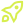Subscribe to our 0800-DEVOPS Newsletter## Not sure where to start? Let our experts guide you. Send us your query through this contact form.## Get in touch## Use the form below to apply for course# Hibernate inheritance mapping

## 07.09.2012

Hibernate offers us three basic ways to map class inheritance to relational database:

1. Table per class hierarchy
2. Table per subclass
3. Table per concrete class

Each of these have it’s strengths and limitations and we will make short review of them and present simple examples based on following class hierarchy:

```public abstract class Animal {
private Long m_id;
private String m_name;
private BigDecimal m_weight;

public Long getId() {
return m_id;
}
public String getName() {
return m_name;
}
public void setName(final String p_name) {
m_name = p_name;
}
public BigDecimal getWeight() {
return m_weight;
}
public void setWeight(final BigDecimal p_weight) {
m_weight = p_weight;
}
}

public class Bird extends Animal {
private BigDecimal m_wingsSpan;

public BigDecimal getWingsSpan() {
return m_wingsSpan;
}
public void setWingsSpan(final BigDecimal p_wingsSpan) {
m_wingsSpan = p_wingsSpan;
}
}

public class Dog extends Animal {
private BigDecimal m_lungsVolume;

public BigDecimal getLungsVolume() {
return m_lungsVolume;
}
public void setLungsVolume(final BigDecimal p_lungsVolume) {
m_lungsVolume = p_lungsVolume;
}
}

public class Fish extends Animal {
}```

1. Table per class hierarchy
In this mapping approach we map whole class hierarchy to one database table. It’s main feature is it’s simplicity, we can read/save our object in just one table and there is no need to multiple create/update sql statements (when we save or update object) or join on multiple tables (when we read object). Main weakness of this approach is that we can’t have NOT NULL constraint on any column mapped to property declared in any of subclasses.

```<class name="Animal" table="ANIMAL">
<id name="m_id" type="long" column="ID" access="field">
<generator>
<param name="sequence">ANIMAL_SEQ</param>
</generator>
</id>

<discriminator column="TYPE"/>

<property name="name" type="string" length="100" column="NAME" not-null="true"/>
<property name="weight" type="big_decimal" column="WEIGHT" not-null="true"/>

<subclass name="Bird" discriminator-value="BIRD">
<property name="wingsSpan" type="big_decimal" column="WING_SPAN"/>
</subclass>

<subclass name="Dog" discriminator-value="DOG">
<property name="lungsVolume" type="big_decimal" column="LUNGS_VOLUME"/>
</subclass>

<subclass name="Fish" discriminator-value="FISH"/>
</class>```

2. Table per subclass
In this mapping approach we map properties contained in base class to one database table and properties contained in each subclass to it’s target table. This way for hierarchy of one base class with three inherited classes we have four tables in database where each of tables mapped to subclass is connected to table mapped to base class by foreign key. Weakness of this approach is that we have more complicated database schema where it is more difficult to follow object in database. Strength of this approach is that we can use NOT NULL constraints to ensure integrity of all properties from subclasses. This is mapping where database structure closely follows object hierarchy.

```<class name="Animal" table="ANIMAL">
<id name="m_id" type="long" column="ID" access="field">
<generator>
<param name="sequence">ANIMAL_SEQ</param>
</generator>
</id>

<discriminator column="TYPE"/>

<property name="name" type="string" length="100" column="NAME" not-null="true"/>
<property name="weight" type="big_decimal" column="WEIGHT" not-null="true"/>

<joined-subclass name="Bird" table="BIRD">
<key column="ANIMAL_ID"/>
<property name="wingsSpan" type="big_decimal" column="WING_SPAN" not-null="true"/>
</joined-subclass>

<joined-subclass name="Dog" table="DOG">
<key column="ANIMAL_ID"/>
<property name="lungsVolume" type="big_decimal" column="LUNGS_VOLUME" not-null="true"/>
</joined-subclass>

<joined-subclass name="Fish" table="FISH">
<key column="ANIMAL_ID"/>
</joined-subclass>
</class>```

3. Table per concrete class
In this mapping approach we map each concrete (non-abstract) subclass to one database table. Therefore we don’t have to have database table for abstract root class and we have reduced number of tables to three and as result we can easily find all data for each concrete object instance in just one place in database. Problem with this mapping is that we have “column duplication” in database (for each field in base class we have column in subclass table).

```<class name="Animal">
<id name="m_id" type="long" column="ID" access="field">
<generator>
<param name="sequence">ANIMAL_SEQ</param>
</generator>
</id>

<discriminator column="TYPE"/>

<property name="name" type="string" length="100" column="NAME" not-null="true"/>
<property name="weight" type="big_decimal" column="WEIGHT" not-null="true"/>

<union-subclass name="Bird" table="BIRD">
<property name="wingsSpan" type="big_decimal" column="WING_SPAN" not-null="true"/>
</union-subclass>

<union-subclass name="Dog" table="DOG">
<property name="lungsVolume" type="big_decimal" column="LUNGS_VOLUME" not-null="true"/>
</union-subclass>

<union-subclass name="Fish" table="FISH"/>
</class>```
##### So, which mapping approach should I use?

Before deciding which mapping to choose we have to take close look at our domain model and distribution of data (fields) through model (classes). One (extreme) case is that all properties are contained in our root class and we have subclasses used only to differentiate types of base class and to define different behavior for each specific type. Another (also extreme) case is when we have base class used only to semantically mark kinship of our classes (and maybe define common behavior) but all data (fields) are contained in subclasses.

First case would be ideal case for “table per class hierarchy” mapping and second case would be ideal for “table per concrete class” or “table per subclass” mapping. Of course, our problem will most often fall somewhere in between these two extremes and we will have to decide which approach should we take by weighting importance of NOT NULL constraint usage in ensuring our data integrity (if any property mapped to subclass is mandatory) versus simplicity of database schema (and relying on application to ensure our data integrity). Rule of thumb would be that for domain model where most data are contained in base class we would prefer to use “table per class hierarchy”, for model where data are evenly distributed through base class and subclasses we would prefer to use “table per subclass” and for model where data are mostly contained in subclasses we would prefer to use “table per concrete class” or “table per subclass”.

Hibernate offers use some additional mapping alternatives and it is possible to use combination of different mapping approaches for same class hierarchy. To get more information on this topic check Hibernate reference documentation.